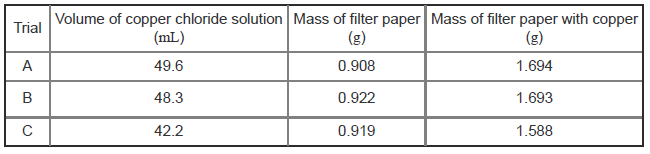# Problem: Part A. Write the empirical formula of copper chloride based on the experimental data.In a student experiment, the empirical formula of a copper halide was found by adding aluminum metal to an aqueous solution of the halide, displacing copper metal. The copper metal was filtered, washed with distilled water, dried, and weighed; three separate determinations were performed. The copper halide solution contained 42.62 g of copper chloride per liter. The student recorded the following experimental data.

###### FREE Expert Solution

We are given with 42.62 g/L of copper chloride in solution and a set of data. Let us add details to the table to get the Mass of Copper filtered and the average of the masses of copper, including the average volume of copper chloride solution:

We find the mass of Copper by subtracting the mass of filter paper from the mass of filter paper with copper.

Trial A

Trial B

Trial C

0.742 g Cu

At the same time, we find the average volume of copper chloride solution.

46.7 mL copper chloride solution

90% (297 ratings)###### Problem Details

Part A. Write the empirical formula of copper chloride based on the experimental data.

In a student experiment, the empirical formula of a copper halide was found by adding aluminum metal to an aqueous solution of the halide, displacing copper metal. The copper metal was filtered, washed with distilled water, dried, and weighed; three separate determinations were performed. The copper halide solution contained 42.62 g of copper chloride per liter. The student recorded the following experimental data.